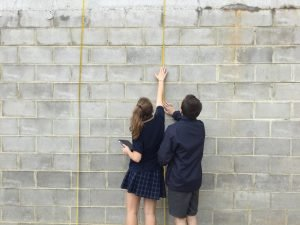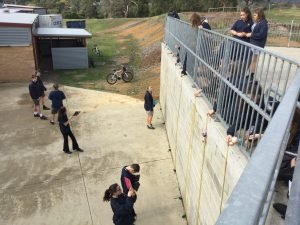# Mathematics

Mathematics provides students with access to important mathematical ideas, knowledge and skills that they will draw on in their personal and work lives. Mathematics is the study of numbers, measurement and geometry, statistics and probability. In mathematics, students will develop mathematical understanding, fluency, reasoning, modelling and problem-solving.

At Yarra Hills we believe learning about maths should be a hands-on journey into an exciting world of patterns and problems. We understand each student comes to us at a different stage of maths understanding and so our classes allow students to access the maths from their unique starting point and from there develop their knowledge and skills. We rely heavily on a wide range of interactive online resources and hands-on class.

Mathematics is meant to help us make sense of our world and give us the tools to work in it. Too often maths classes are about answering questions in a textbook that has little to do with the maths we experience in everyday living. We have worked hard to create a maths curriculum that focusses on maths that is real, rich and relevant.

Real
Our students use maths to investigate and solve problems faced by real people in our society. For example,

• students construct their own inclinometers and then use them to take measurements of buildings, using trigonometry to calculate angles and lengths (civil engineers)
• students conduct surveys in the community and analyse the results comparing them to national surveys carried out by the Australian Bureau of Statistics (statisticians)
• students investigate the coverage of a liquid and then calculate the amount of liquid required to “paint” a building (painters)

Rich
Many of our activities are open ended allowing students to dig deeper into the problems exploring the maths involved. Rather than talk about a problem, students are encouraged to take a hands-on approach and experiment with possible solutions.

Relevant
We use maths everyday in many aspects of our lives. Students also use maths both inside and outside of school and we encourage our students to develop the ability to see the links between maths inside the maths classroom and maths in other subjects and in other aspects of life.

We make extensive use of technology in our classes using a wide range of applications to help us understand and use maths. We have also built up a library of maths tools from trundle wheels to giant decks of cards to help our students investigate and experiment. We do not use a set textbook as these meet the requirements of very few students and are limited to a narrow range of abilities. Instead we make use of an enormous wealth of material found online and created by our own teachers that allow students at all levels of ability to learn and grow in their maths understanding and give them a solid foundation for real life and later studies in mathematics.## VCE Maths

Yarra Hills Secondary College currently offers the following Maths subjects at VCE:

• Maths Methods
• General Maths
• Further Maths

We encourage all of our students to consider taking a maths subject in VCE as many courses require maths and many employers are looking for students with evidence of good maths skills. As a result, over 85% of our VCE students select a maths subject in VCE.

Our students have gone on to work in maths-related fields such as:

• astrophysics
• research science
• architecture
• accounting
• maths teaching